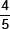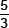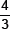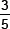# Constructions-Sample Papers

Class IX Math
Test for Construction
Total Marks: 40
Total Time: 60 mins
1.   Draw a line AB = 12 cm and divide it in the ratio 3 : 5. Measure the two parts.
2.   Construct a triangle similar to a given triangle ABC with its sidesth of the corresponding sides of ΔABC. Also, write the steps of construction.
3.   Draw a circle of radius 3 cm. Take a point at a distance of 5 cm from the centre of the circle. Measure the length of each tangent.
4.   Divide a line segment of length 6 cm internally in the ratio 3 : 2.
5.   Construct a triangle similar to a given ΔABC with sides equal toof the corresponding sides of ΔABC.
6.   Construct a ΔPQS such that PQ = 4.5 cm, PS = 4 cm and SQ = 5.4 cm. Construct another triangle P’QS’ similar to ΔPQS with side S’Q = 7.5 cm.
7.   Draw a right triangle in which the sides (other than hypotenuse) are of length 4 cm and 3 cm. Then construct a triangle similar to it and of scale factor.
8.   Draw a triangle ABC with side BC = 7 cm, ∠B = 45°, ∠A = 105°. Then, construct a Δ whose sides aretimes the corresponding sides of ΔABC.
9.   Draw a circle of radius 1.5 cm. Take a point P outside it. Without using the centre, draw two tangents to the circle from the point P?
10.   Construct a triangle ABC, in which AB = 5 cm, BC = 6 cm and AC = 7 cm. Then construct another triangle whose sides aretimes the corresponding sides of ΔABC.